# Cardinality and the axiom of choice

If the axiom of choice is false, then there are two sets which are not the same size, but neither one of them is larger than the other. This, and similar seemingly absurd results, are sometimes used as motivation for the axiom of choice. But this is not so absurd when you unpack what it means: A set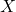is said to be at least as large as a set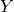if there is an injection frominto, the same size asif there is a bijection betweenand, and strictly larger thanif it is at least as large asbut not the same size. So all this result is saying is that if the axiom of choice is false, then there are two sets, neither of which can be injected into the other. It’s not hard to find two groups without an injective homomorphism between them in either direction, and not hard to find two topological spaces without any injective continuous maps between them in either direction. So why not sets?

The usual reason to think this shouldn’t happen for sets is that cardinalities of sets are supposed to correspond to an intuitive notion of size. But this intuitive interpretation is not God-given; it is an interpretation that people came up with because it seemed to fit. If the axiom of choice is false, this interpretation fits less well. The axiom of choice can be viewed as saying that sets are so flexible that the possibility of fitting one inside another is limited only by their relative sizes, whereas the negation of the axiom of choice says that sets have some essential structure that can’t be adequately interpreted as size.

But it gets worse. Without the axiom of choice, it’s possible to have an equivalence relationon a setsuch that there are strictly more equivalence classes ofthan there are elements of. For instance, if all subsets ofare Lebesgue measurable, then this can be done with an equivalence relation on, namelyiff.

Surely this is still absurd? Again, I think it is not. It only sounds absurd because of the inappropriate language we used to describe the situation in which there’s an injection fromto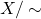but no bijection between them. Instead, you can think about it asandbeing flexible in the ways that allowto fit inside, but rigid in ways that preventfrom fitting inside, rather than in terms of bigness.

I suspect that, to many people, “has strictly larger cardinality than” sounds more absurd than “andhave incomparable cardinalities” does, but it really shouldn’t, since these are almost the same thing. The reason these are almost the same is that an infinite set can be in bijection with the union of two disjoint copies of itself, another phenomenon that could be thought of as an absurdity if the identification of cardinality with size is taken too literally, but which you have probably long since gotten used to. Ifandhave incomparable cardinalities andcan be put into bijection with two copies of itself, then, using such a bijection to identifywith two copies of itself, mod out one of them bywhile leaving the other unchanged. The set of equivalence classes of this new equivalence relation looks like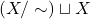, whicheasily injects into.

And it shouldn’t be surprising that there could be an equivalence relationsuch thatcan’t inject into; the only obvious reasonshould be able to inject intois that you could pick an element of each equivalence class, but the possibility of doing this is a restatement of the axiom of choice. For instance, in the example ofand, a set consisting of one element from each equivalence class would not be Lebesgue measurable, and thus doesn’t exist if all sets are Lebesgue measurable.

The sense in which cardinality can be about structure more general than size can become even more apparent in more austere foundations. Consider a mathematical universe in which everything that exists can be coded for somehow with natural numbers, and every function is computable. There’s a set of real numbers in this universe, which we know of as the computable real numbers: they’re coded by numbers representing programs computing Cauchy sequences that converge to them. It doesn’t really make sense to think of this universe as containing anything “bigger” than, since everything is coded for by integers. But Cantor’s theorem is constructive, so it applies here. Given a computable sequence of computable reals, we can produce a computation of a real number that isn’t in the sequence. So the integers and the (computable) reals here have different “cardinalities” in the sense that, due to their differing computational structure, there’s no bijection between them in this computability universe.

I think that it can be helpful to think of cardinalities as potentially being about inherent structure of sets rather than simply “size” even if you’re assuming the axiom of choice the whole time. Fun fact: if there’s any model of ZFC at all, then there’s a countable model. This often strikes people as absurd; ZFC asserts the existence of uncountable sets, like, so how could something countable be a model of ZFC? The answer is that an infinite set being uncountable just means that there’s no bijection between it and. A countable model of ZFC can contain a countable set but not contain any of the bijections between it and; then, internally to the model, this set qualifies as uncountable. This is sometimes described as the countable model of ZFC “believing” that some of its sets are uncountable, but being “wrong”. I think this is a little sloppy; models of ZFC are just mathematical structures, not talk radio hosts with bad opinions. Restricting attention to a certain model of ZFC means imposing additional structure on its elements; namely that structure which is preserved by the functions in the model. This additional structure isn’t respected by functions outside the model, just like equipping a set with a topology imposes structure on it that isn’t respected by discontinuous maps.

To give a couple concrete examples of how I visualize cardinality as being about structure: When we encounter mathematical objects of cardinalityin practice, they often naturally carry separable topologies on them, so I think ofas being thicker than, but no longer. Since smaller ordinals are initial segments of larger ordinals, I think of, the cardinality of the first uncountable ordinal, as longer than, but no thicker.being well-orderable would mean we can rearrange something thick into something long.

It’s interesting to note that you can forge something long () out of something thick () by modding out by an equivalence relation. (This gives another example of a quotient ofthat, axiom of choice aside, it’s perfectly reasonable to think shouldn’t fit back inside). This is becauseis the cardinality of the set of countable ordinals, each countable ordinal is the order-type of a well-ordering of, and well-orderings onare binary relations on, aka subsets of. So, starting with(with cardinality), say that any two elements that are both well-orderings of the same order-type are equivalent (and, if you want to end up with just, rather than, also say that all the left-overs that aren’t well-orderings are equivalent to each other). The set of equivalence classes then corresponds to the set of countable ordinals (plus whatever you did with the leftovers that aren’t well-orderings).

The idea behind this post was a specific instance of the general principle that, when a result seems absurd, this doesn’t necessarily refute the foundational assumptions used to prove it, but rather means that your way of thinking isn’t well adapted to a mathematical universe in which those assumptions are true. Another example of this is that the Banach-Tarski theorem and similar results often strike people as patently absurd, but people get used to it, and one could try explaining why such results shouldn’t be seen as as absurd as they first seem, as a way of conveying intuition about what a mathematical universe in which the axiom of choice holds looks like.

While I don’t find the allegedly counterintuitive things that are likely to happen without the axiom of choice compelling, this doesn’t undercut other arguments for the axiom of choice. I think the strongest is that every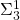statements (a broad class of statements that arguably includes everything concrete or directly applicable in the real world) that can be proved in ZFC can also be proved in ZF, so assuming the axiom of choice isn’t going to lead us astray about concrete things regardless of whether it is true in some fundamental sense, but assuming the axiom of choice can sometimes make it easier to prove something even if in theory it could be proved otherwise. This seems like a good reason to assume the axiom of choice to me, but that’s different from the axiom of choice being fundamentally true, or things that can happen if the axiom of choice is false being absurd.